×
Get Full Access to FSU - CHM/B 4053 - Study Guide - Midterm
Get Full Access to FSU - CHM/B 4053 - Study Guide - Midterm

×

FSU / Chemistry / CHM/BCH 4053 / Subcellular organization such as organelles.

# Subcellular organization such as organelles. Description

##### Description: This is a comprehensive study guide for exam 1 in Biochemistry 1. IT includes information directly from lecture slides and information given from the professor, as well as side notes and help videos
23 Pages 7 Views 7 Unlocks
Reviews

Biochemistry Exam 1 Study Guide ­ BCH

## Subcellular organization such as organelles.Lecture 1: An Introduction to Biochemistry

Why do we study biochemistry?

∙ Biochemistry principles guide biological processes in all organisms including the  humans.

∙ We can use biochemistry to study a variety of human pathogens in other organisms,  such as the prion disease (proteinaceous pathogen)

o This pathogen is also seen in sheep and involves a similar protein ∙ Biochemistry is used to study and treat cancer ­ How do anticancer drugs work? o Examples of anticancer drugs:

∙ Alkylating agents If you want to learn more check out Who was first to observe microorganisms, most likely protozoa/bacteria?

∙ Topoisomerase inhibitors

∙ Antimitotic agents

∙ RNA/DNA antimetabolites

Biochemistry basics:

Cells are the Smallest Units of Life

∙ Cells are the smallest units of life.   Don't forget about the age old question of How are domestic and transnational terrorism related?

∙ A cell is capable of growth, replicating, energy transforming and metabolizing. ∙ All living cells fall into two distinct classifications:

## Thermodynamics deals with systems at equilibrium.o Prokaryotic: single celled; lack nuclei ­ includes Archaea [which has some  eukaryotic characteristics] Don't forget about the age old question of What is allotropes?

o Eukaryotic: multicellular; contains organelles (e.g. mitochondria) ∙ Various types of cells (specialization)

∙ Cells share a common biochemistry:

o Genes that code for enzymes for essential biological processes are conserved  between some bacteria and humans!

o Living organisms are highly organized and consist of biomolecular hierarchy ∙ Atoms ­ molecules ­ larger molecular assemblies

Cell Structures ­ Differences between prokaryotes and eukaryotes

∙ Both have biological membranes that are extremely important. Both have DNA (genetic  material)

∙ Note that prokaryotes have a single, circular DNA structure where eukaryotes  have multiple, “strandlike” DNA that is organized into chromosomes.

## Biochemistry principles guide biological processes in all organisms including the humans.∙ Prokaryotes: no subcellular organization

∙ Prokaryotes do not have structured or membrane bound organelles like  eukaryotes.

∙ Eukaryotes: subcellular organization such as organelles

∙ Eukaryotes have many organelles such as mitochondria, nucleus, peroxisome, etc.

Chemistry of biomolecules:

∙ Biomolecules, or macromolecules, are composed of carbon. Carbon outer shell electrons have three possible hybridization states which allow them to form three kinds of covalent  bonds with other atoms: We also discuss several other topics like  Linked to what types of crime?
If you want to learn more check out what is Current Account Balance?

o Sp3 ­ single bonds If you want to learn more check out What are the effects of hydroxy groups?

 These bonds are 25% s properties, 75% p properties

o Sp2 ­ forms double bonds

 These bonds are 33% s bond, 66% p bond

o Sp ­ triple bonds

 These are 50% s bond and 50% p bond

o Various hybridizations change the properties of the molecules.

∙ Limited sets of possible combinations of C, H, O, P, S and N atoms are found in  biomolecules.

∙ Biomolecule precursors are water (H2O), carbon dioxide (CO2), and inorganic nitrogen  compounds (NH4+, NO3­, N2);

o The nitrogen is produced predominantly from bacteria and plants.  o Precursor molecules are built into slightly bigger molecules called metabolites. ∙ Biomolecules are constructed from several simple molecules according to a hierarchy of  structure complexity.

∙ Precursors are converted to metabolites which mediate cellular energy transformation and biosynthesis of various sets of building blocks (amino acids, sugars, nucleotides, fatty  acids and glycerol)

∙ Macromolecules serve functional purposes:

o Macromolecule building blocks ­ macromoles

∙ Amino acids ­ proteins

∙ Nucleotides ­ nucleic acids (DNA/RNA)

∙ Fatty acids ­ lipid molecules

Bonding and chemistry of biomolecules

∙ Hydrogen bonds will be extremely important in biochemistry! Lone pairs can form these hydrogen bonds.  These bonds define the specificity of the molecule.

Biomolecule Directionality ­ all large molecules are made with directionality. ∙ Peptides ­ peptides construct proteins

o Proteins are important for taking the building blocks and building the  macromolecule.  Proteins have a variety of functions in the cell, and do most of the  “work” (and use most of the “energy)

∙ Proteins are important enzymes in biological processes such as the genesis of ATP and the contraction of muscle.

∙ Proteins can also be used for movement of vesicles, changing

cell/organelle composition, etc.

∙ Carbohydrates

o This is our major source of energy and ATP for the body.

o Carbohydrates can be used in post­translational modification (glycoprotein) and  the identification of viruses and bacteria (i.e. gram positive/negative coat).  ∙ Nucleic acids

o Goes from the 5' to the 3'direction (this is how the nucleotide is read) o Human DNA has 3 billion such nucleotides, with a matching pair!  o RNA will have a hydrogen group at the 3', where a DNA will have an OH at the 3'

Features of macromolecules:

∙ Macromolecules are synthesized from building blocks and have a sense of  directionality (important for three dimensional architecture);

∙ Macromolecules are informational (important for replication).

∙ Biomolecules have characteristic three­dimensional architecture ­ structural functional  relationship is extremely important!

o STUCTURE IS DIRECTLY RELATED TO FUNCTION.

Bonds/Intermolecular forces in biochemistry:

∙ Weak forces include hydrogen bonding, van der Waals forces, ionic interactions and  hydrophobic interactions (noncovalent interactions). These forces maintain biological  structure and determine biomolecular interactions.

o Van der Waals interaction ­ ANY pair of atoms will feel this interaction ∙ This is a “spontaneous dipole” brought about by the natural charges on  molecules.

o Hydrophobic interaction ­ interactions that arise from molecules trying to "avoid"  water interaction

∙ These molecules do not interact with water.

∙ These are nonpolar interactions, along with these molecules being in  water.

∙ Hydrophilic interactions are the opposition to hydrophobic interactions.  o Why are these called weak forces? Covalent bonds are much stronger than  noncovalent bonds (which biomolecular interactions are)

∙ We are talking of interactions between molecules, or folding of molecule  itself.

∙ Weak forces also mediate biomolecule recognition (e.g. antibodies and bacteria) ∙ Structural complementarity (similar to in that in lock­and­key or pieces of a puzzle)  determines biomolecule interaction.

Water as a solvent:

∙ Think about all of our molecules (in this class) existing in water.

∙ Water is capable of forming hydrogen bonds (partial overlapping of electrons) ∙ Water is clathrate (consisting of lattice that can trap other molecules) ∙ It is capable of dissolving ions. This is due to the slight negative and positive charge of  water (polarization)

∙ Water is amphipathic = nonpolar + polar

∙ Properties of Water:

∙ Chemical formula: H2O

∙ Its shape (or structure) determines its function. Water is good solvent for ionic  substances (salt), nonionic but polar (sugar, alcohols, amines, aldehydes and  ketones);

∙ Water is extremely good at shielding the electrostatic interactions.

∙ Dielectric Constant D: The ability of water to surround ions in dipole  interactions in order to diminish their electrostatic attractions is a measure of its  dielectric constant, D. The larger D is, the smaller the Columbic force between the negative and positive ions is: F = e1e2/Dr^

∙ The further they are the weaker it is, the closer they are the stronger it is. ∙ This is the measure of how effectively the water will shield the

electrostatic attractions

∙ This depends on the property of our solvent. Pure water is 80.

∙ H­bonds: H­bonds occur between polar groups. The oxygen in water is a good H bond acceptor and hydrogens are good H­bond donors. Thus water can form H bonds with many polar groups (I.,e, C=O, NH3, OH);

∙ This ordering process costs energy

∙ Hydrophobic interactions: When non­polar  molecules are dissolved in water, water molecules reorganize to form a cage­like structure  (clathrate) that surrounds each solute molecule. This process is accompanied by significant  ordering of the solvent or negative entropy. Under these conditions, non­polar molecule  experience a net attraction for one another that is called hydrophobic interaction. In another word, two nonpolar molecules have a tendency to stay close together in water.

Lecture 2: Water, Acids and Bases

Today we are adding things to water that are dissociable:

∙ Protons dissociate in water H+ or H3O+

∙ The ability to dissociate ions in water determines hydrostatic potentials, electric bonding  and the relationships between different groups in biological systems.

Chemical Equilibrium (review)

∙ For a general reaction A + B = C + D, the four components will eventually reach a state  at which the amount of each component no longer changes.  This state is called  equilibrium.  One can define the equilibrium constant, Keq, as Keq = [ A] eq [ B] eq [C] eq [ D] eq

o Equilibrium: no net change over time of the reactants or products.  (this does not  mean there is no chemical reaction, there is just no NET CHANGE).

∙ Note that the subscript "eq" under each concentration denotes concentrations only  measured at chemical equilibrium.You do not need to memorize the numbers. This is simply an example.  You will need to know  how to calculate equilibrium constants, and the definition of equilibrium.

The pKa measures the intrinsic ability for glucose (or any molecule) to be phosphorylated by  hexokinase (or any other reactant/catalyst):

∙ Given the same temperature and molecules, you will ALWAYS reach the same  concentration.

∙ It is a property of ATP and glucose.

o It depends on the glucose charge and structural properties.

Dissociation constant (review)

∙ For a dissociation process where one reactant is water, A + H2O = C + D another  constant called dissociation constant, Ka is defined as Ka = Keq[H2O} = [C] eq[ D] eq [ A] eq

o Molar: 1 mole per 1 liter

o The subscript a comes from the term "acid".  If it is a base, it is Kb.  o Because water concentration is very high, this remains to be constant. ∙ As one can see, the larger Ka value is, the harder it is for reactants to associate.  Note also that the unit of Ka is molar.Once again, you do not need to know exact values, just principles.

Ionization of water:

∙ Autodissociation: Water conducts electricity through dissociated ions: H20 = H+ + OH­ ∙ Note in reality: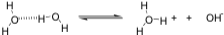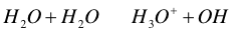∙ What we will do next is to calculate the dissociation constant of water according to its  pure chemical equilibrium.

 Hydroxide and proton concentrations are 10^7 M (note this would yield a 7 pH)  Calculating the dissociation constant, including the 55.5 M value for water  concentration, gives you 1.8*10^16 M (if you would like to see the whole calculation, it is on her slide set).

o Where Kw is defined as the ion product of water, Kw = [H+][OH­} = 10^14  M^2.  Ka is a constant because the ions have negligible influence on water concentration,  or Kw = Keq [H2O][H2O} = constant.

o MORAL OF THE STORY: The dissociation constant for water is small, thus  water does not have a tendency to dissociate.

 Subnote: Kw is 10^­14 for water no matter what.

pH (measures the amount of hydronium ions)

∙ pH values of a solution is defined as pH = log [H+]

o Example: For pure water, pH = log [H+] = log [10^­7] = 7

o pH is a measure of molarity and therefore, concentration

∙ For convenience, define pOH = log [OH] thus:

o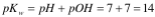o The pKw will be the same for water even if I put it in water!

∙ You should be able to use dissociation constants where dissociation of hydronium ion is  defined as 1, that is, water has equal tendency to dissociate H+ and OH­ ions:  o What is a strong acid? As soon as it is in water, it nearly completely dissociates ­  there is vitually none left.

∙ Strong acids will give up ALL their protons.

∙ Its Ka value is very high.

 Examples: HCl: 10^7M; H2SO4: 10^2 M

o What is a strong base? Aw soon as it is in water, it nearly completely dissociates ∙ Strong bases will take all the protons from water, leaving OH­.

∙ Its Ka vlues are very low (and Kb is very high!)

 Examples: H3PO4: 7.5 *10^3 M; HCO3­: 4.8*10^­11M

∙ What happens in water? How does this affect the solution?

o For acids: HA +H2O = H3O+ + A (the conjugate base)

∙ If we put HCl in solution, the pH will drop significantly, due to an  increase in the concentration of hydronium ions.

o For bases: B + H2O = HB (Conjugate acid) + OH

Calculate pH Values:

∙ Strong acids and strong bases: full dissociation!

o If we put .01M of HCl in solution, we will have .01M of hydronium ions in the  solution.

∙ By taking the negative log, we see a pH of 2 for 0.01 M HCl

∙ Weak acids dissociate the same as strong acids, but do not completely dissociate.  To  calculate the Ka of weak acids, you must take the exact concentrations and plug them into  the equation: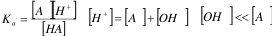Henderson­Hasselbalch Equation:

∙ HH equation relates pH value to dissociation constant and concentrations of ions.  o In biological solutions, we use another form pH equation (Henderson Hasselbalch)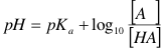o This is another form of the dissociation constant of acid (Ka).  The derivation for  the Henderson­Hasselbalch equation from the dissociation constant of acid is:  ∙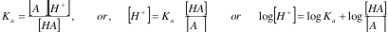∙ The dissociation constant can be manipulated, giving us an equation for  hydronium ions.  If we take the log of both sides, we get the Henderson

Hasselbalch equation.

∙ We know that pH = log[H+], and pKa = log Ka, giving us pH = pKa + log  [A]/[HA]

∙ The pH is the environmental variable in the body.

∙ Note on HH equation:

o Fraction of charged vs. noncharged can change under different pH. or the charged species is influenced by the amount of H+ (or pH) in solution;

o Charged/noncharged = 1 when pH=pKa;

o In general, Charged/noncharged=10(pH­pKa)

Lecture 3: Titrations

Titrations:

∙ Titrations are used to determine the amount of acid in a solution by adding bases.  ∙ There are two equilibria occurring in solution when an acid is added to water o HA = A­ + H+; strong dissociation (large Ka)

o H+ + OH­ = H2O; strong association (small Ka)

o Titration is forcing the acid to dissociate until it is completely gone.   ∙ When a base, typically NaOH, is slowly added to the solution, the hydroxyl ion will  neutralize any hydronium ion present by equilibrium (2).  More acid will be dissociated and the aid will eventually dissociate completely (to A­).

o By monitoring the pH value (via a pH meter), you can determine the acid  concentration in a solution (quantitatively).

∙ The solution pH is related to the amount of hydroxyl ion according to the HH equation  (note that when [A­] = [HA}, pH = pKa, because the log of the ratio would be O) o The HH is a measure of the ratio of [A­] and [HA], aka how much is  dissociated.

o You can plot this as the function of pH vs. the concentration of OH­.  Your curve  will be the "titration curve"

∙ Titration curves:

o When [OH­] << 0.5, the solution is in the completely acidic form. o When [OH­} = 0.5, the acid form = the conjugated base, or the neutral form =  negatively charged form

∙ pKa = pH here.

o When [OH­] >>> 0.5, the solution is in the completely conjugated base form  (negatively charged)

∙ Note: titration curves will be extremely important when we get to pI in amino acids!

How to draw a titration curve:

1 Exam the molecule first. Find out the number of dissociable protons;

2 Obtain the pKa value(s) for all dissociable group(s);

3 Start the graph by labeling the vertical axis “pH” and the horizontal axis “equivalent OH­”; 4 Find the values of pKa on the vertical axis and mark them;

5 Find the value of 0, 0.5, and 1 on the horizontal axis and mark them; (You may need to  mark 1.5, 2.0 etc. as well. Depending on the number of dissociable groups) 6 Draw two intercepting lines, one through the first pKa value, the other through the 0.5  equivalent OH­ position as shown below (repeat for all pKas):

Protonation:

∙ The concept of titration can be applied to any ionizable group on the molecule.  The  graph shown below are titration curves for acetic acids, imidazole, and phosphoric acid. ∙ Multiple ionizable groups have different pKas, and pose a problem! o Note: An acid can have pKa>7! (e.g. HPO4)

o The lower the pH value, the more protonated the species will be (especially if it  has multiple ionizable groups)

∙ Example:

o Group 1 has a pKa of 5, and group 2 has a pKa of 7.

o Group 1 is a stronger acid and more likely to lose its proton.

Buffers:

∙ Buffers are solutions that resist changes in pH as external acids or bases are added.  IT  is important to maintain a pH required for the body physiologically; for instance, blood pH  is 7.4 , a gastric juice pH is 1;

∙ The buffer chosen such that pH = pKa, where the titration curve is almost flat ∙ Commonly used buffers:

o Tris (8.1), MES (6.1), PIPES (6.8), MOPS (7.2) and HEPES (7.5)

Determining the charge of a titrated molecule:

1 Exam the molecule first. Find out the number of dissociable protons;

2 Calculate the charge of the molecule with ALL DISSOCIABLE PROTONS attached, q0; 3 At equivalent [OH­] = 0, the total charge = q0;

4 At equivalent 0< [OH­]<1, (q0­1)<total charge< q0;

5 At equivalent [OH­]=1, total charge = q0­1;

6 If the molecule contains more than one dissociable protons, you will continue to titrate, and the charge of the molecule will further decrease;

7 At equivalent 1<[OH­]<1.5, (q0­2)<total charge< (q0­1);

8 At equivalent [OH­]=2, total charge = q0­2.

Keep in mind how to determine the pH and pKa of dissociable groups.

Lecture 4: Thermodynamics

NOTE: I will be using G often in place of delta G, as well as H in place of delta H,  and S in place of delta S.

Introduction:

∙ Thermodynamics studies principles that describe the flow and exchange of heat, energy, and matter in system of interests;

∙ Thermodynamics deals with systems at equilibrium;

o What is Gibbs free energy and how does it relate to the equilibrium constant? Is a  particular reaction favorable or not?

∙ Thermodynamics principles determine if a system is stable or if a reaction can occur  spontaneously;

o Thermodynamics talks about the flow and exchange in systems. These are  multiple molecule systems.

∙ A thermodynamics system can be one of

1  Isolated ­ No exchange of either energy or matter;

2 Closed ­ No exchange of matter, may exchange energy;

3 Open ­ may exchange energy or matter

∙ Living systems are normally open systems;

o Most reactions that we look at are not independent of each other.  They are  constantly exchanging material and matter.

Thermodynamics:

∙ Treat biological systems as abstract systems;

∙ Thermodynamics principles determines the direction of biological processes ∙ Provide quantitative measures to biological systems

Thermodynamic laws:

The first law: conservation and conversion

∙ For a system that has converted from state 1 to state 2 (no matter what path it took), its  internal energy is the sum of heat absorbed from outside and work done to the system:  o E=E2 ­ E1 = q + W

o E = internal energy, can exchange with surrounings in the form of heat and work;  q = absorbed heat from outside; W = work done to the system.

∙ How do we understand work?

o Work takes various form such as electrostatic, mechanical, or heat.  o Example: air compression: W = P (V2 *V1) = PV

∙ A more useful function for biological system, enthalpy H is defined as H = E  +PV.

 H is the energy that is put into your removed from a system during

a process. If we have a negative H value, a process is endothermic

(energy is put off).  If a process has a positive H value, it is

endothermic (requires energy)

∙ H can be measured experimentally.

∙ Enthalpy directly measures heat absorbed by the system under constant  pressure.

The Second Law: direction of a thermal process

∙ Systems tend to proceed from ordered (low entropy or low probability) states to  disordered (high entropy or high probability) states;

o Entropy is the measure of randomness or disorder ina  system

∙ The entropy of the system plus surroundings is unchanged by reversible processes; the  entropy of the system plus surroundings increases for irreversible processes; o Entropy of the universe is ALWAYS increasing!

∙ All naturally occurring processes proceed toward equilibrium, to a state of minimum  potential energy

∙ Entropy, S, is a quantity that measures the order of a system and can be quantitatively  expressed as S=klnW, where W is the number of ways of arranging a system without  changing its energy and k is Boltzmann’s constant (k=1.38x10­23 J/K);

o You do not need to know the equation, only the principle.

o High entropy is more disorder.  Low entropy is more order.

o This particular isolated system is always trying to maximize the entropy. HELP VIDEO: https://www.youtube.com/watch?v=axG9HuqViDY

The third law: absolute zero cannot be reached.

∙ The third law states that entropy of an ordered substance must approach zero as the  temperature approaches 0 K.  This law provides bases for quantifying entropy for any  substance if the heat capacity, Cp, can be evaluated as: S = C d ln T.

o You do not need to know this derivation, just the concept.

∙ Gibbs free energy, G is defined as:

o G = H ­ TS

o This is enthalpy minus the quantity of temperature and entropy.

o Gibbs free energy determines the spontaneity or likeliness of a reaction.  Will a  reaction happen?

o Where G1 is the before energy and G2 is the energy after a reaction, G2 ­ G1 is  the G value.

o G indicates the direction of a chemical reaction:

∙ Endergonic, unfavored G >0

 Systems tend to stay in lower energy states.  Here, G1 has the

lower energy state, so it is unlikely it will move to G2.

∙ Exergonic, favored G< 0

 Here, G2 has a lower energy state than G1, so it is more likely to

move to G2, producing product.

∙ Equilibrium G = 0

o G is related to equilibrium constant, Keq for an equation A + B = C + D as: ∙ G = G0 + RT ln [C][D]/[A][B]

∙ Note: there is no subscript eq for these equations!

 Aka, this calculation can be done at any concentration

∙ Where G0 is the standard state of free energy change (1 M solution)

∙ R = gas constant = 8.314 j/mol*K

o At equilibrium G = 0o Note that Keq and G0 are intrinsic properties or a particular reaction, thus  equations (2) and (3) can always be used for a particular reaction, but G depends on  the concentrations.

o The thermodynamic definition of equilibrium is that there is no change in  concentrations.  IF the total difference in G is 0, G2 = G1.

o Influence of pH on G0 at a standard state, [H+] = 1M, pH = 0! Because nothing is buffered at pH = 0, sometimes G0 is used.o G0 is NOT impacted by changes in concentration.

Free energy, enthalpy and entropy:

∙ Free energy change is due to changes in either enthalpy or entropy: o G = H ­ TS

∙ Example: Dissolving ions in H2O. H2O molecules rearrange themselves around ion, thus  more ordering. This is an unfavored process due to negative entropy. But the favored  molecular interactions make enthalpy decrease which results in a total Gibbs free energy  decrease making the overall process favored.

o Is the solvation of an ion in water favorable?

o Before the reaction we have free ions and water itself.

∙ We have to add up the gibbs free energy of these reactions.

∙ Before ions are put in here, water forms a network of hydrogen bonds due  to the lone pair on the oxygen and the protons.

o After solvation, the water will arrange itself around the positive ion.  The water  will arrange itself with a less degree of freedom (they can only orient one way). ∙ The reactants have a higher entropy

∙ Before the ion comes in, we have hydrogen bonds, which are favorable  interactions.  These have a low enthalpy (low internal energy).

∙ The ionic interactions with water are exteremly favorable (electrostatic  interactions) giving you a low, low, low enthalpy.

o THIS PROCESS IS FAVORABLE! (due to low change in enthalpy, nd a low  change in entropy).

The next lecture talks about the application of thermodynamics and entropy, along with  enthalpy.

NOTES GIVEN BY THE PROFESSOR AT THE END OF THE POWERPOINT: (She never  actually talked about these or went over them)

∙ Thermodynamic laws are useful for bulk systems such as a solution (rather than a single  object), In another word, thermodynamic principles are developed to deal with large  ensemble of molecules. it helps us to know what makes a certain reaction move the  direction it does, and what is the fundamental properties that describe the equilibrium;

∙ We will discuss in a more abstract way the thermodynamic principles and their  applications on specific biochemical reactions in solutions.

∙ When one considers a system, one has to consider its interaction with environment.  Environment is described by several environment variables such as T, P, V, and N.  Thermodynamic descriptions of a system comprise of a set of these variables. Not all  environmental variables are independent of each other. Depending on which of the T, P, V,  N are kept at constant, different thermodynamic descriptions are used for the system. For

biological systems (constant T, V but variable N, or called Grant canonical ensemble in  statistical mechanics), Gibbs free energy, (G), H, S make the best set.

∙ Let’s start with, E, the internal energy of the ensemble. E consists of two parts, one  potential energy (how strongly molecules interact) and one kinetics energy (how fast  molecules move in solution). Thus E depends on the structure of the molecules and is  proportional to temperature of the ensemble.

∙ First law says that E is conserved and its change equals to q+W _­­­perpetual machines  are impossible;

∙ H is defined because for reactions occur in solution, the change in H can be directly  measured.

∙ H = E + PV   So DH = DE + D(PV) = q+w+d(PV) = q + (­PDV) + P(DV), so H is just  the heat absorbed at constant P, very easy to measure, and it reflects the internal energy of  the system

∙ Second law introduces the concept of entropy. One can have a system that completely  equal in internal energy but in different arrangement; this cost energy, so simply using E,  one can not judge whether a system is at equilibrium. A new quantity called S is  introduced, which describes the order of the system.

∙ Second law says that for a closed system, entropy is maximized. Or a spontaneous  process in a closed system moves to maximal entropy.

Lecture 5: Application of Gibbs Free Energy

Applications of Gibbs Free Energy:

∙ Knowing initial concentrations of reactions and products, total Gibbs free energy change,  DG, can be calculated. It depends on concentrations of reactants and products ∙ We can use these values to determine the spontaneity of glycolysis.  o We can determine that glycolysis has a negative delta G, and can move forward.

Coupled reactions: one particular reaction may be favorable.  This reaction is paired with a non favorable reaction to drive it to completion.

∙ Biological systems are open systems, and they can exchange energy and matter, thus can  be coupled.

o Although the first reaction may not be favored (reaction 1 ­ not favored),  coupling of reaction 2 with reaction one may be favorable (reaction 1+2 ­  favored)

o One example of a coupled reaction is glycolysis.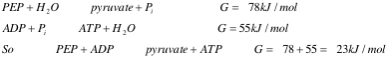∙ The first reaction (with PEP and H2O as reactants) are favored (negative  G)

∙ The second reaction (ADP and P1 as reactants) is NOT favored (positive   G)

∙ If this reaction is coupled, the overall reaction is exergonic.  Biological  enzymes can facilitate the coupling of reactions.

∙ One protein molecule will grab both molecules in one active site and the  product is one spontaneous reaction.

 Here the net production of ATP is favorable.

High energy molecules: the nature of this energy

∙ Virtually all life forms depend on the sun which converts chemical energies to high  energy molecules which then drive endergonic reactions.

o  Examples of these high energy molecules include Phosphoenolpyruvate, 3’,5’­ Cyclic adenosine monophosphate,1, 3­Bisphophoglycerate, acetyl­coenzyme A. ∙ TP also captures this chemical energy.

∙ What is the chemical explanation for this energy? Gibbs free energy ­  looking for high entropy change and low enthalpy change.

o We will study the structure and functions of these molecules in later lectures. ∙ Why can high energy molecules store energy?

o There are three reasons:

∙ destabilization due to electrostatic repulsion (enthalpy increases) ∙ Products contain multiple isomerization (entropy increase)

∙ Resonance stabilization (entropy increase)∙ This structure will eventually need to be memorized, but not right now.  ∙ Note: the energy is stored in phosphoric anhydride linkage. ADP and Pi should be  lower gibbs free energy than ATP.

o Chemically it turns out the phosphate is partially positive.  The positive center  makes the attack of ATP or ADP not very favorable (high enthalpy ­ higher internal  energy)

o The partial positives create repulsion.

o After breaking down the ATP to ADP, this releases energy, lowering the  enthalpy.

∙ Entropic terms: why is the breakdown of these molecules more entropically favored?  o The broke down molecule has higher entropy than the constructed form, due to more ways of arranging the molecule.

∙ Note that there are 5 dissociable protons on ATP.  It thus has a complicated equilibrium  involving hydrolysis.  The highest pKa is 6.95, thus at pH higher than this, ATP is  completely deprotonated.

∙ The effect of concentration on free energy of hydrolysis of ATP ­

o G0 for ATP breakdown is ­30.5.  At standard state, this is a favorable reaction at  pH 7.

o Assume that the average adult human consumes 11,700 kJ per day. Given that  each ATP yields about 50 kJ of free energy, this amounts to about 64 kg ATP  molecules, close to the weight of a human body!

∙ Luckily, our body has higher efficiency and can recycle ATP molecules  after each use. The actual amount of ATP in a human body is only about 50 g.  o Metabolism is complicated because we recycle this ATP/ADP

Lecture 6: Amino Acids!

This playlist of videos can be extremely helpful in inderstanding the basics of amino acids  covered in this lecture: https://www.youtube.com/watch?

v=KIvBn6gfRgY&list=PLbKSbFnKYVY3GmRofoqKNtmB2_PckhrLX

Structure of amino acids:

∙ Amino acids are building blocks for proteins. There are total of 20 naturally occurring  amino acids;

∙ Each amino acid contains an amino, a carboxyl group and a side chain group. The side  chain gives the identity of the amino acid.

∙ Amino acids can be classified by polarity of their side chains.

o The pKa value of the amino group is much higher, meaning at physiological pH it will be protonated, where the pKa of carboxyl group yields a deprotonated group. o Remember that pKa is an intrinsic property of functional groups.

o You MUST be able to recognize an amino acid based on this structure: ∙Memorize your amino acids!

∙ Nonpolar amino acids: you have 8 of them

o I find it helpful to break these up into “simple, short” amino acids (alanine, valine, leucine, isoleucine, proline and “complicated/cyclic” amino acids (methionine,  phylalanine, trypotophan).

oo These side chains are nonpolar due to their hydrocarbons ­ they cannot accept a  proton or have charge interactions.

o The two cyclic side chains

o Note the importance of proline: proline is the only amino acid with a ring  incorporated into the amine group.  Becaue of this, it has very limited mobility.  It can cause “kinks” in the structure of the protein, and is common in the turns of beta  sheets.

o Note that Isoleucine is just a modified form of leucine.  Methionine includes a  sulfur molecule.

∙ Polar, uncharged amino acids: you have 7

o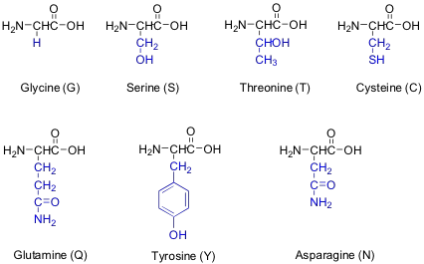o Note that asparginine and glutamine are the uncharged forms of glutamic acid and aspartic acid

o Note the prevalence of oxygen in the side chains (serine, threonine, glutamine,  tyrosine, asparginine).  This is a good indicator of polar, uncharged.

o Note the sulfur on cysteine.  Due to this sulfur, cysteine can form disulfide bonds,  and has a different chirality than all other amino acids.

o Note glycine is the smallest of the amino acids.  It is NON­CHIRAL.  ∙ Polar, charged amino acids: there are 5

o ∙ Note: Histidine, lysine, arginine all contain nitrogenous side chains.  These all have  positive side chains and will have distinctive titration curves.

∙ Glutamic acid and aspartic acid are formed simply by taking glutamate or aspartate and  switching an alcohol group (OH) in place of the NH group.

Note: I found these videos extremely helpful in memorization of amino acids!  https://www.youtube.com/watch?v=gq­rWb0fmzQ

One of the best ways to study is simply to draw them, and focus on their properties.  Put  them in different groups, make your own neumonics, make flashcards, and PRACTICE!

Important amino acid “fun facts”:

∙ Certain amino acids and their derivatives are biochemically important.  ∙ For example, the visible symptoms of allergies are caused by the release of histamine in  mast cells, a type of cell found in loose connective tissue. Histamine dilates blood vessels,

increases the permeability of capillaries (allowing antibodies to pass from the capillaries to  surrounding tissue), and constricts bronchial air passages.

o The molecular mechanism of histamine function is by its specific binding to a  protein called histamine H1 receptor.

∙ Serotonin, which is derived from tryptophan, function as neurotransmitters and  regulators.

Optical activity and stereochemistry of amino acids:

∙ All amino acids but glycine are chiral molecules.

∙ There are two possible configurations around Ca that constitute two non­superimposable  mirror image isomers, or enantiomers.

∙ Enantiomers display optical activity in rotating the plane of polarized light. All natural  amino acids are L­ isomers (as opposed to D­isomers).

∙ Optical activity/chirality examples:

o

UV­absorbing properties of Amino acids:

∙ Only three amino acids, Phe, Tyr, and Trp, absorb light in the near UV range (230 nm 300 nm). These amino acids dominate the UV absorption spectra of proteins.  ∙ The wavelength maxima for tyrosine and tryptophan are around 280 nm.  ∙ In contrast, nucleic acids (we will discuss later) have absorption maximum of 260 nm.  ∙ A simple UV scan can allow one to distinguish between protein and nucleic acids.

Lecture 7: Titrating Peptides and Peptide Purification

Typical titratable groups on amino acids: be familiar with the titratable groups! ∙

∙ Notice, the nitrogen based groups tend to be basic (high pKa), where the carboxylic  side chains (such as on glutamic acid and aspartic acid) are acidic (low pKa). ∙ Sulfur side chains: disulfide bonds are extremely important in protein folding! We have  lots of cysteines in our side chains.

∙ Tyrosine side chains have protons that are difficult to remove.

Amino Acids are weak polyprotic acids:

∙ Each amino acid contain dissociable protons.  The degree of dissociation of these proteins depends on the solution pH;

∙ First consider acid­base behavior of glycine, the simplest amino acid. There are two  ionizable groups.

∙ Titration is used to dermine how much acid we have in a solution:

o When we have multiple ionizable groups, the titration curve looks a little  different.

o For uncharged amino acids, the pKa1 is 0.5.  This is when we have an equal  amount of zwitterion and cation.

o A zwitterion is an energetically neutral form of the amino acid, where we have a  deprotonated carboxylic acid group, and a protonated amine group.

o When we are at one equivalent, we have all zwitterions!

o When we are titrating to 2 equivalent, we will have all the glycine in the anion  form (completely deprotonated, with a charge of ­1).

o When we are half way between one equivalent and two equivalents, we will  have equal amounts of zwitterion and anions.

pI: a pI value for an amino acid is defined as the pH value at which the amino acid is neutral.  In  the case of glycine (and other noncharged amino acids):

∙ pI=1/2(pKa1+pKa2)

∙ This is basically the "average" pKa for the amino acid.  Here we have all neutral ions.   For uncharged amino acids, all the amino acids in the solution are zwitterions.  For charged amino acids, note, this is NOT purely zwitterions.

∙ The pH value of the solution is related to different charge species of glycine by: o

o Where [gly0] is the concentration of zwitterion.

o When you are close to a second pKa, the values of the first pKa is negligible.

Ionaization of side chains:

∙ Polar amino acids contain additional dissociable protons, their titration curves are more  complex.  However, the same principles apply.  Lets look at titration of glutamic acid. o Here we have 3 pKa values!

o pKa1 is for the main chain carboxyl group, pKa2 is for the side chain carboxyl  group and pKa3 is the for amino group. The pI for glutamic acid is between pKa1 and  pKa2

o You MUST know how to calculate all pI values for any given amino acid, if you  have the pKa values! Simply take the average of all the values.

o For acidic amino acids, you will have a pI lower than 7 (normally around 2­4).   For basic amino acids, you will have pI higher than 7 (normally around 9­11).  For  neutral amino acids, the pI will be around 7.

∙ Sample calculation:

o What is the pH of a lysine solution if the side chain amino group is 3/4  dissociated?

o There are three titrable groups on lysine, we will illustrate this in class. The  sidechain starts to ionize when pH> pKa3=10.5.

∙ pH = 10.5  + log/ ­ (Henderson­Hasselback)

 When we are 3/4 dissociated, 3 parts are dissociated, and 1 part is  not dissociated. That is where the / comes from.

∙ pH = 10.5 + (0.477)

∙ pH = 10.977 = 11.0

∙ Why does the same carboxyl group have different pKa values?

o Recall that pKa is related to the dissociation constant, Ka.

o The equilibrium of proton dissociation from a chemical group is influenced by its  chemical environment. Thus the dissociation constant of carboxyl group is different  when it is in different chemical environment.

o For instance, when it is near a positively charged group, say, lysine, the carboxyl  group is more likely to dissociate its proton because the negatively charged  carboxylate interacts with the positively charged lysine. Thus its Ka value is higher,  thus pKa will be lower. The same argument can be made for the main chain carboxyl  group because it is near an amino group.

o Remember that pKa = log Ka

Separation and analysis of amino acids

∙ All separation methods are based on physical (size) and chemical (charge) properties of  amino acids.

∙ We will focus on the method called ion­exchange chromatography o Beads, or resins that absorb negatively charged amino acids are called anion  exchangers; the container itself is positively charged.

o Beads, or resins that absorb positively charged amino acids are called cation  exchangers; The container itself is negatively charged.

o For example, if you are trying to track lysine, you will use a positively charged  container.  After you trap the molecule, you use a competing method to elute the  molecule.

o You use salts. For example, sodium will be attracted to the negative column, and  will compete with the lysine.  Here the lysine will begin to come off and seep down  the container.

∙ Mixture of amino acids can be loaded on ion­exchange columns at low salt and are  separated by flowing through increasing amount of salts.

∙ WE can use a UV absorption spectrum. The red line on a graph indicates the ionic  strength (more molecule competition).

o For example, aspartic acid will come out very early, followed by serine and  lysine.

Lecture 8: Chemical Properties of a peptide bond

Lecture 8 ­ WHAT ARE WE SKIPPING (what really matters, you don’t have to study this!! :D) ∙ Everything from slide 5 to the end.

∙ Protein sequence determination

Introduction to proteins:

∙ Proteins are of vital importance of biological systems because of:

o Their ability to interact with other molecules based on 3D structures; o Their ability to catalyze chemical reactions.

Proteins are linear polymers of amino acids:

∙ Terminology:

o Residues­­­­amino acids

o Dipeptide­­­a peptide containing two amino acids

o Polypeptide­­­a peptide containing more than a dozen amino acids o Monomer ­­­­­a protein containing a single polypeptide

o Multimers­­­­­­a protein containing more than one polypeptide

o Homodimer­­­­­a protein contain two identical polypeptide

o Heterodimers­­­­a protein contain two different polypeptide

o Tetramers­­­­­­a protein containing four polypeptides (can be homo­ or hetero­)  o The primary structure of DNA is the exact amino acid composition in order.  ∙ For a polypeptide of N amino acids, there is 20N possible amino acid sequences. Thus  there is virtually unlimited number of amino acid sequences

Protein sequence (primary structure):

∙ The amino acid sequence of a protein

o is a unique characteristic of the protein

o is encoded by the nucleotide sequence of DNA, is thus a form of genetic  information

o is read from the amino terminus to the carboxyl terminus

Structure of a peptide bond:

∙ Two amino acids are joined by the peptide bond, a reaction catalyzed by the enzyme  called ribosome in all cells:

∙ Due to the double bond character, the six atoms of the peptide bond group are always  planar! ­ the double bonds cannot rotate. Due to resonance structure, the first C alpha and  the next carbon are always planar.